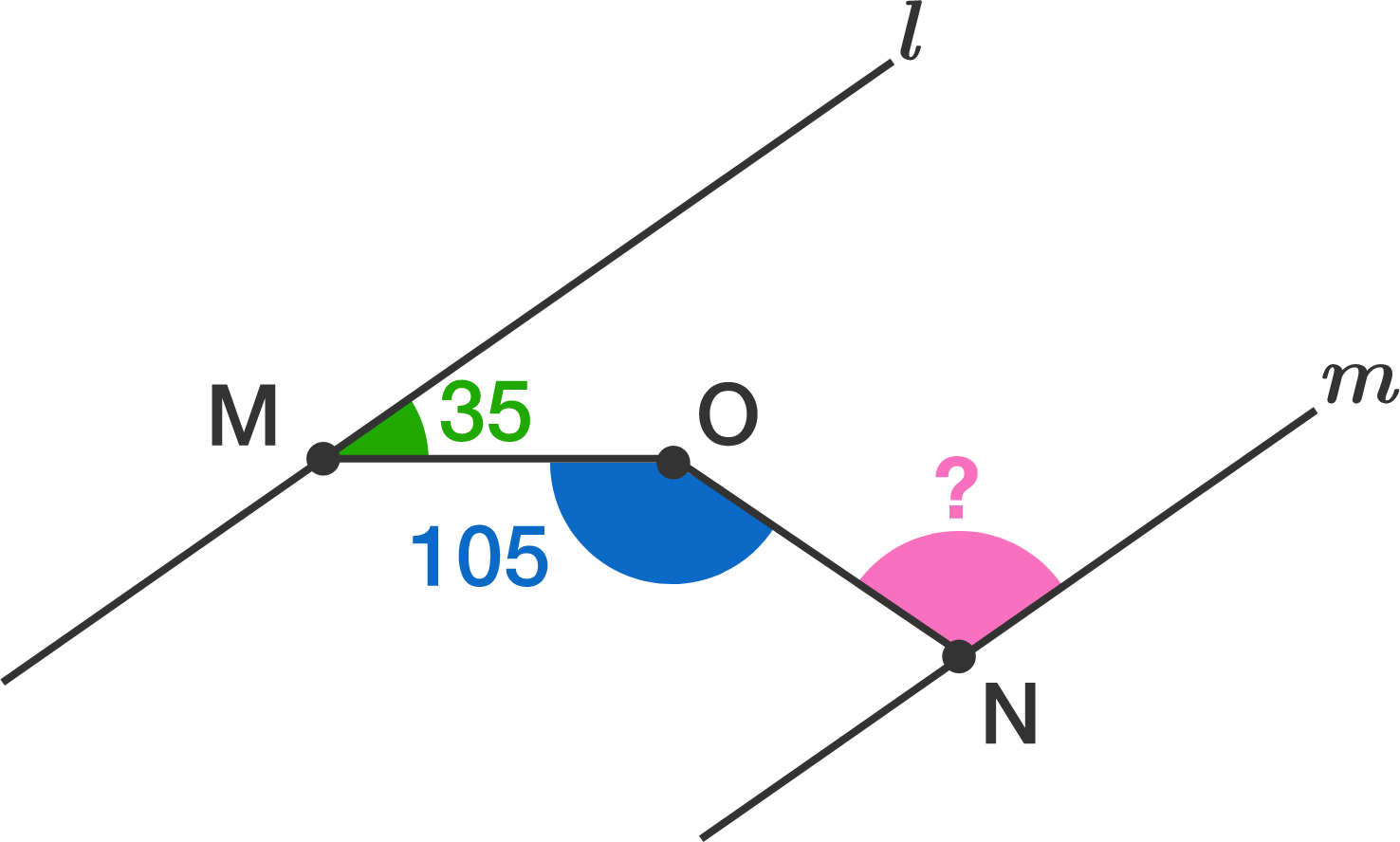# Where Are The Alternate Angles?

Geometry Level 1Lines $l$ and $m$ are parallel. $M$, $N$ and $O$ are points such that $M$ is on $l$ and $N$ is on $m$ and $O$ is between the two lines. If the smaller angle between $l$ and $MO$ is $35^{\circ}$ and $\angle MON = 105^{\circ}$, find the acute angle between $m$ and $NO$.

Clarification: The diagram is not accurate

×